# Mid Point Formula

Mid Point Formula is a foundational concept in coordinate geometry and it plays a crucial role in finding the midpoint of a line segment. There are instances in Coordinate Geometry where we need to know the mid-point of two given points or the mid-point of a line segment, where the midpoint formula comes in handy as it is a simple and effective way to calculate the midpoint of any given line segment, regardless of its length or position on the coordinate plane. This article covers the Mid Point Formula in detail, with its derivation using the similarity of triangles. Also, covers the sample problems so that students can grasp the concept with some practice at hand.

## Mid Point Definition

The point which divides the line exactly in two equal halves is the midpoint of the line. In other words, the ratio of both halves of the line in which the midpoint divides it is 1:1.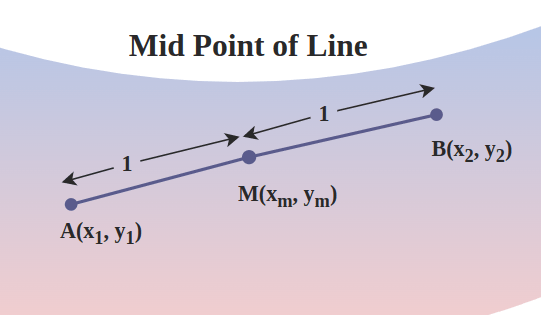## Mid Point Formula

For a line segment AB in Cartesian coordinate where the x-axis coordinate of point A is x1 and the y-axis coordinate of point A is y1 and similarly, the x-axis coordinate of point B is x2 and the y-axis coordinate of point B is y2, the mid-point of the line will be given by (xm, ym).

The formula for the mid-point  (xm, ym) is,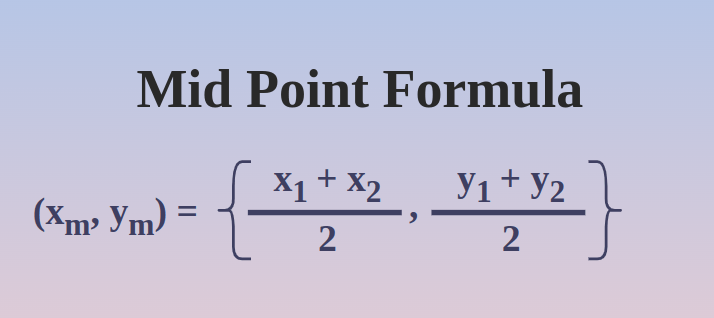## Derivation of Mid Point Formula

Let P(x1,y1) and Q(x2,y2) be the two ends of a given line in a coordinate plane, and R(x,y) be the point on that line which divides PQ in the ratio m1:m2 such that

PR/RQ = m1/m . . .(1)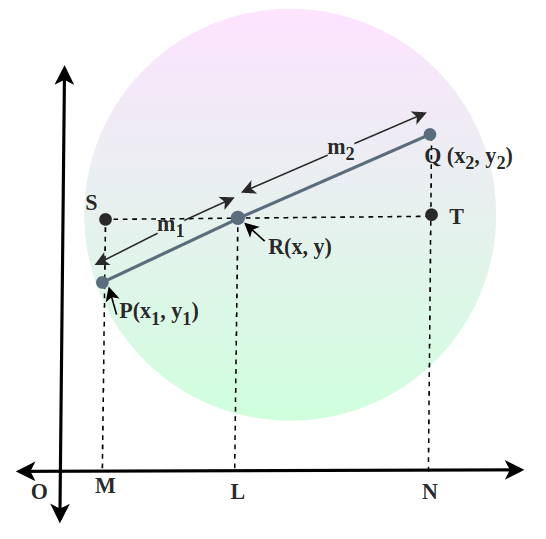Drawing lines PM, QN, and RL perpendicular on the x-axis and through R draw a straight line parallel to the x-axis to meet MP at S and NQ at T.

Hence from the figure, we can say,

SR = ML = OL – OM = x – x1 . . . (2)

RT = LN = ON – Ol = x2 – x . . . (3)

PS = MS – MP = LR – MP = y – y1 . . . (4)

TQ = NQ – NT = NQ – LR = y2 – y . . . (5)

Now triangle ∆SPR is similar to triangle ∆TQR,

Therefore,

SR/RT = PR/RQ

By using equations 2, 3, and 1, we know,

x – x1 / x2 – x = m1 / m2

⇒ m2x – m2x1 = m1x2 – m1x

⇒ m1x + m2x = m1x2 + m2x1

⇒ (m1 + m2)x = m1x2 + m2x1

⇒ x = (m1x2 + m2x1) / (m1 + m2)

Now triangle ∆SPR is similar to triangle ∆TQR,

Therefore,

PS/TQ = PR/RQ

By using equations 4, 5, and 1, we know,

y – y1 / y2 – y = m1 / m2

⇒ m2y – m2y1 = m1y2 – m1y

⇒ m1y + m2y = m1y2 + m2y1

⇒ (m1 + m2)y = m1y2 + m2y1

⇒ y = (m1y2 + m2y1) / (m1 + m2)

Hence the coordinates of R(x,y) are,

R(x, y) = (m1x2 + m2x1) / (m1 + m2), (m1y2 + m2y1) / (m1 + m2)

As we had to calculate the midpoint, therefore, we keep the values both of m1 and m2 as same i.e.

For the mid-point we know by the definition of mid-point, m1 = m2 = 1

(x, y) = ((1.x2 + 1.x1) / (1 + 1), (1.y2 + 1.y1) / (1 + 1))

x, y = (x2 + x1) / 2, (y2 + y1) / 2

## How to find Mid Point?

To find the coordinates of the midpoint of any given line segment we can use the midpoint formula if the endpoints of the line segment are given. Consider the following example for the same.

Example: Find the coordinates of the midpoint of a line segment whose endpoints are (5, 6) and (-3, 4).

Solution:

As we know, the midpoint of a line segment is given by the formula:

Midpoint = ((x1+x2)/2 , (y1+y2)/2)

where (x1, y1) and (x2, y2) are the coordinates of the endpoints of the line segment.

Midpoint = ((5+(-3))/2, (6+4)/2)

⇒ Midpoint = (2/2, 10/2)

⇒ Midpoint = (1, 5)

Therefore, the coordinates of the midpoint of the line segment are (1, 5).

## Related Formula

There are similar formulas to the midpoint formula, which are as follows:

• Section Formula
• Centroid Formula

### Section Formula

Section Formula is used to find the coordinate of the point which divides the given line segment in the desired ratio. Let us assume the endpoints of a line segment are A and B with coordinates (x1, y1) and (x2, y2), and P be the point that divides the line segment joining the line AB in m:n. Then coordinate of P is given by

P(x, y) = [(mx2 + nx1)/(m+n) , (my2 + ny1)/(m+n)]

### Centroid Formula

The Centroid formula is used to find the center point of polygons and mathematically for triangles and quadrilaterals is given as follows:

### Centroid of a Triangle Formula

The coordinates of the centroid of a triangle with vertices (x1, y1), (x2, y2), and (x3, y3) are:

C(x, y) = ((x1 + x2 + x3)/3, (y1 + y2 + y3)/3)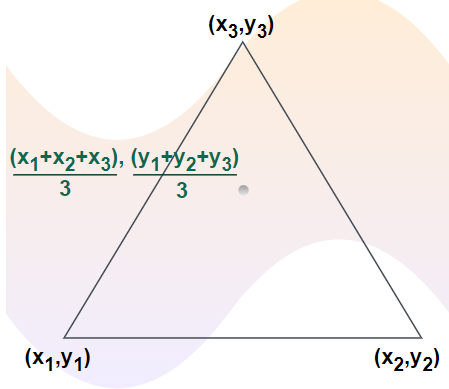### Centroid of a Quadrilateral Formula

The coordinates of the centroid of a quadrilateral with vertices (x1, y1), (x2, y2), (x3, y3), and (x4, y4) are:

C(x, y) = ((x1 + x2 + x3+ x4)/4, (y1 + y2 + y3+ y4)/4)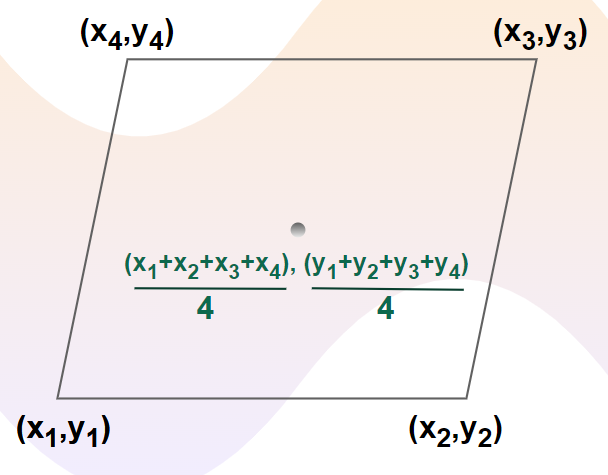## Sample Problems on Mid-Point Formula

Problem 1: What is the mid-point of line segment AB where point A is at (6,8) and point B is (3,1)?

Solution:

Let the midpoint be M(xm,ym),

xm = (x1 + x2) / 2

x1 = 6, x2 = 3

Thus, xm = (6 + 3) / 2 = 9 / 2 = 4.5

ym = (y1 + y2) / 2

y1 = 8, y2 = 1

Thus, ym = (8 + 1) / 2 = 9 / 2 = 4.5

Hence the midpoint of line AB is (4.5, 4.5).

Problem 2: What is the mid-point of line segment AB where point A is at (-6,4) and point B is (4,2)?

Solution:

Let the midpoint be M(xm,ym),

x1 = -6, x2 = 4, y1 = 4, y2 = 2

(xm, ym) = ((x1 + x2) / 2, (y1 + y2) / 2)

(xm, ym) = ((-6 + 4) / 2, (4 + 2) / 2)

(xm, ym) = ((-2) / 2, (6) / 2)

(xm, ym) = (-1, 3)

Hence the midpoint of line AB is (-1, 3).

Problem 3: Find the value of p so that (–2, 2.5) is the midpoint between (p, 2) and (–1, 3).

Solution:

Let the midpoint be M(xm, ym) = (-2, 2.5) where,

x1 = -1, xm = -2

y-coordinate of the end point is already known as 2, hence we need to find only the x-coordinate

xm = (x1 + x2) / 2

-2 = (-1 + p) / 2

-4 = -1 + p

p = -3

Hence the other end-point of the line is (-3, 2).

Problem 4: If the coordinates of the endpoints of a line segment are (3, 4) and (7, 8), find the distance between the midpoint of the line segment and the point (3, 4).

Solution:

Let A(3, 4) and B(7, 8) be the endpoints of the given line segment, and C is the midpoint of line segment AB.

Then using the midpoint formula,

Coordinate of C = ( (3+7)/2 , (4+8)/2 ) = (5, 6)

Using Distance Formula

Distance = √{(x2 – x1)2 + (y2 – y1)2}

⇒ Distance = √{(3 – 5)2 + (4 – 6)2}

⇒ Distance =√{(-2)2 + (-2)2}

⇒ Distance =√8 = 2√2

Therefore, the distance between the midpoint of the line segment and point (3, 4) is 2√2.

## FAQs on Mid Point Formula

### Q1: What is the Mid-Point Formula?

Mathematically Midpoint Formula is given as follows:

Midpoint = ((x1 + x2)/2 , (y1 + y2)/2)

Where (x1, y1) and (x2, y2) are the coordinates of the two endpoints of the line segment.

### Q2: What is the Significance of Mid-Point Formula?

The mid-point formula is significant because it allows us to find the center point of any line segment on a Cartesian coordinate system.

### Q3: What are Some Applications of Mid-Point Formula?

There are many use cases of the mid-point formula as in geometry we can use it for solutions and properties of triangles, polygons, and other shapes, in physics it has application in finding the center of mass as well.

### Q4: Can the Mid-Point Formula be used for Three or More Points?

No, the mid-point formula can’t be used for three points as mid-point is defined for only two point. For three points we can use centroid formula if we want to find the coordinate of centroid for the triangle formed by the given three points.